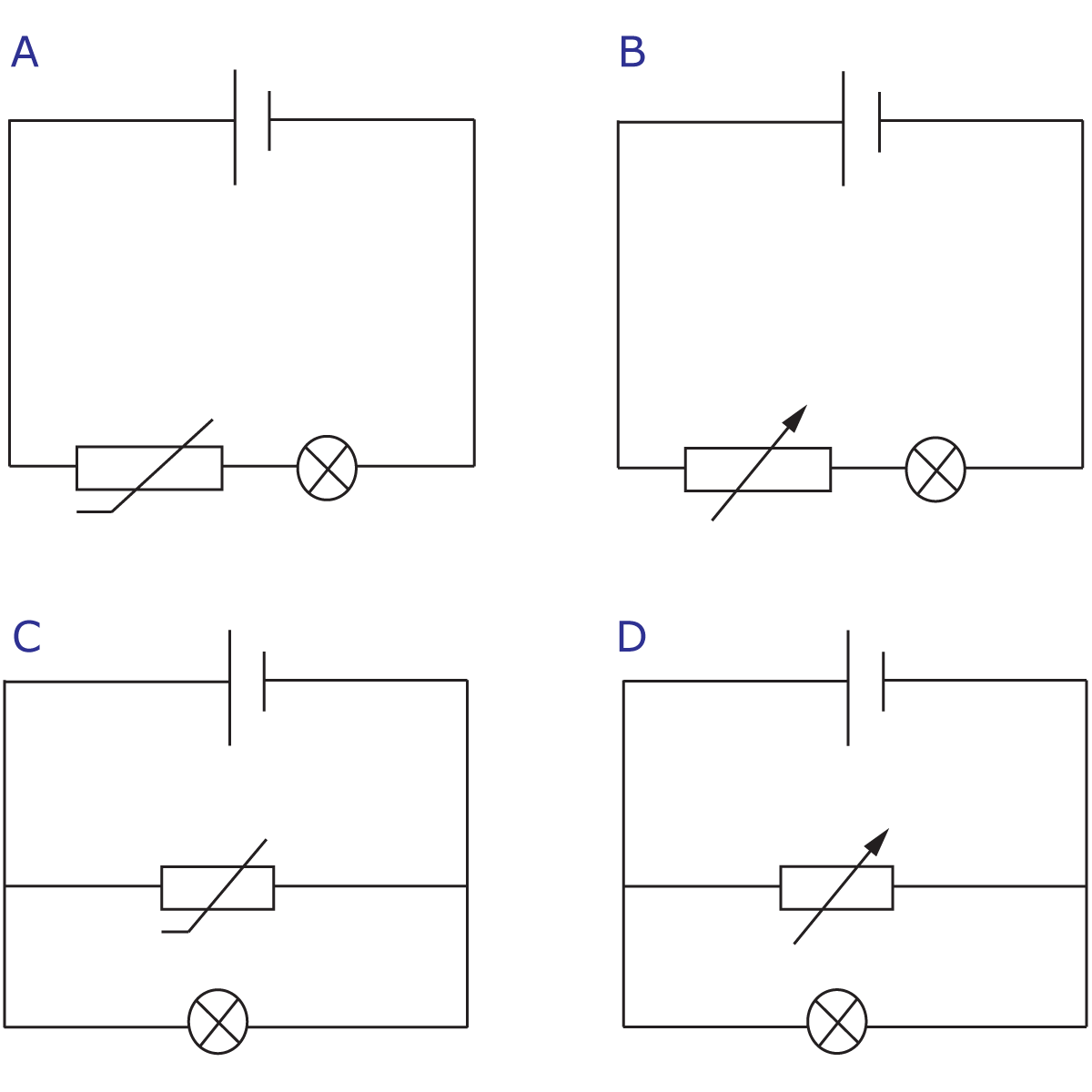10 minutes maximum! Can you do it in 5?

Q1-3. Can you identify these components?

1.2.3.4. A component that emits light and only lets current pass through one way is called

• A. an L.D.R.
• B. an L.E.D.
• C. a diode
• D. a thermistor
5. Which component has a voltage(V) ~ current (I) graph like this?• A. a resistor
• B. a diode
• C. a bulb (filament lamp)
• D. an L.D.R.
6. Which component has a voltage(V) ~ current (I) graph like this?• A. a resistor
• B. a diode
• C. a bulb (filament lamp)
• D. an L.D.R.

7. Two components are frequently used as sensors for heat and light . They are.....

For Sensing Heat For Sensing Light
A
LDR LED
B
Thermistor LED
C
LDR Thermistor
D
Thermistor LDR

8. A variable resistor is used to vary the brightness of a bulb. Which circuit correctly shows the right components and the right circuit layout?9. When a current flows through any resistor, some of the electrical energy is transfered to ..

• A. heat energy.
• B. chemical energy.
• C. friction.
• D. static electricity.
10. This circuit has a 'light sensor' component and a bulb.On a sunny day ...

• A. the bulb gets dimmer as the light sensor resistance increases.
• B. the bulb gets dimmer as the light sensor resistance decreases.
• C. the bulb gets brighter as the light sensor resistance increases.
• D. the bulb gets brighter as the light sensor resistance decreases.